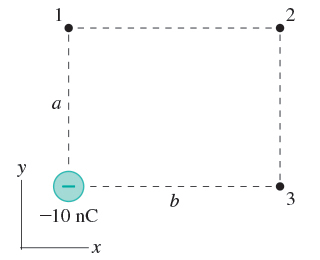# Problem: 1) What is the electric field at point 1 in (Figure 1)? Assume that a= 3.0 cm and b = 4.0 cm.2) What is the electric field at point 2 in (Figure 1)?3) What is the electric field at point 3 in (Figure 1)?

###### FREE Expert Solution

The electric field due to a point charge is given by:

$\overline{){\mathbf{E}}{\mathbf{=}}\frac{\mathbf{k}\mathbf{q}}{{\mathbf{r}}^{\mathbf{2}}}}$, where r is the distance from the point charge q.

97% (19 ratings)###### Problem Details

1) What is the electric field at point 1 in (Figure 1)? Assume that a= 3.0 cm and b = 4.0 cm.

2) What is the electric field at point 2 in (Figure 1)?

3) What is the electric field at point 3 in (Figure 1)?Frequently Asked Questions

What scientific concept do you need to know in order to solve this problem?

Our tutors have indicated that to solve this problem you will need to apply the Electric Field concept. You can view video lessons to learn Electric Field. Or if you need more Electric Field practice, you can also practice Electric Field practice problems.

What professor is this problem relevant for?

Based on our data, we think this problem is relevant for Professor Haddad's class at Colorado School of Mines.